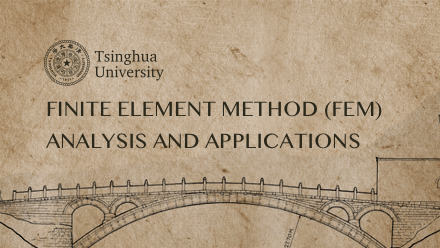# Finite Element Method (FEM) Analysis and Applications

The main content of this course includes: fundamental mathematics and mechanics method, basic properties of the finite element method

## Finite Element Method (FEM) Analysis and Applications课程简介：前往报名学习## Finite Element Method (FEM) Analysis and Applications课程简介：

The main content of this course includes: fundamental mathematics and mechanics method, basic properties of the finite element method

## Finite Element Method (FEM) Analysis and Applications课程目录：

#### 0、Course summary

--Finite element, infinite capabilities

#### 1、Introduction

--1.1 Classification of mechanics：particle、rigid body、deformed body mechanics

--1.2 Main points for deformed body mechanics

--1.3 Methods to solve differential equation solving method

--1.4 Function approximation

--1.5 Function approximation defined on complex domains

--1.6 The core of finite element: subdomain function approximation for complex domains

--1.7 History and software of FEM development

--Discussion

#### 2、Finite element method of bar system based on direct stiffness method

--2.1 Principles of mechanic analysis of springs

--2.2 Comparison between spring element and bar element

--2.3 Coordinate transformation of bar element

--2.4 An example of a four-bar structure

--2.5 ANSYS case analysis of four-bar structure

--Discussion

#### 3、Mechanical description of deformed bodies with complex geometry 1

--3.1 Mechanical description and basic assumptions for deformed body

--3.2 Index notation

--3.3 Thoughts on three major variables and three major equations

--3.4 Construction of equilibrium Equation of Plane Problem

--3.5 Construction of strain-displacement relations for plane problems

--3.6 Construction of constitutive relations for plane problems

--3.7 Two kinds of boundary conditions

-- Discussion

#### 4、Mechanical description of deformed bodies with complex geometry 2

--4.1 Discussion of several special cases

--4.2 A complete solution of a simple bar under uniaxial tension based on elastic mechanics

--4.3 The description and solution of plane beam under pure bending

--4.4 Complete description of 3D elastic problem

--4.5 Description and understanding of tensor

--Discussion

#### 5、Principle of trial function method for solving mechanical equations of deformed body

--5.1Main method classification and trial function method for solving deformed body mechanics equation

--5.2 Trial function method for solving pure bending beam: residual value method

--5.3How to reduce the order of the derivative of trial function

--5.4 The principle of virtual work for solving plane bending beam

--5.5 The variational basis of the principle of minimum potential energy for solving the plane bending

--5.6 The general energy principle of elastic problem

--Discussion

#### 6、Classic implementation and finite element implementation based on trial function method

--6.1 Classic method and finite element method based on trial function

--6.2 Natural discretization and approximated discretization in finite element method

--6.3 Basic steps in the finite element method

--6.4 Comparison of classic method and finite element method

--Discussion

#### 7、Finite element analysis of bar and beam structures

--7.1 Construction and MATLAB programming of bar element in local coordinate system

--7.2 Construction and MATLAB programming of plane pure bending beam element in local coordinate syste

--7.3 Construction of three-dimensional beam element in local coordinate system

--7.4 Beam element coordinate transformation

--7.5 Treatment of distributed force

--7.6 Case Analysis and MATLAB programming of portal frame structure

--7.7 ANSYS case analysis of portal frame structure

#### 8、Finite element analysis of continuum structure (1)

--8.1 Two-dimensional 3-node triangular element and MATLAB programming

--8.2 Two-dimensional 4-node rectangular element and MATLAB programming

--8.3 Axisymmetric element

--8.4 Treatment of distributed force

--8.5 MATLAB programming of 2D plane rectangular thin plate

--8.6 Finite element GUI operation and command flow of a plane rectangular thin plate on ANSYS softwar

--Discussion

#### 9、Finite element analysis of continuum structure (2)

--9.1 Three-dimensional 4-node tetrahedral element and MATLAB programming

--9.2 Three-dimensional 8-node hexahedral element and MATLAB programming

--9.3 Principle of the isoparametric element

--9.4Numerical integration

--9.5 MATLAB programming for typical 2D problems

--9.6 ANSYS analysis case of typical 3Dl problem

--Discussion

#### 10、Basic properties in finite element method

--10.1Node number and storage bandwidth

--10.2 Properties of shape function matrix and stiffness matrix

--10.3 Treatment of boundary conditions and calculation of reaction forces

--10.4 Requirements for construction and convergence of displacement function

--10.5C0 element and C1 element

--10.6 Patch test of element

--10.7 Accuracy and property of numerical solutions of finite element analysis

--10.8 Error and average processing of element stress calculation result

--10.9 Error control and the accuracy improving method of h method and p method

--Discussion

#### 11、High-order and complex element

--11.1 1D high-order element

--11.2 2D high-order element

--11.3 3D high-order element

--11.4 Bending plate element based on thin plate theory

--11.5 Sub-structure and super-element

#### 12、Introduction to the application of finite element analysis (1)

--12.1 Finite element analysis for structural vibration: basic principle

--12.2 Case of finite element analysis for structural vibration

--12.3 Finite element analysis for elastic-plastic problems: basic principle

--12.4 Finite element analysis for elastic-plastic problems: solving non-linear equations

--Discussion

#### 13、Introduction to the application field of finite element analysis (2)

--13.1 Finite element analysis for heat transfer: basic principle

--13.2 Case of finite element analysis for heat transfer

--13.3 Finite element analysis for thermal stress problems: basic principle

--13.4 Finite element analysis for thermal stress problems: solving non-linear equation

--Discussion

#### 14、Project

--2D problem: finite element analysis of a 2D perforated plate

--3D problem: meshing control of a flower-shaped chuck

--Modal analysis of vibration: Modal analysis of a cable-stayed bridge

--Elastic-plastic analysis: elastic-plastic analysis of a thick-walled cylinder under internal pressur

--Heat transfer analysis: transient problem of temperature field during steel cylinder cooling process

--Thermal stress analysis: temperature and assembly stress analysis of truss structure

--Probability of structure: Probabilistic design analysis of large hydraulic press frame

--Modeling and application of methods: Modeling and analysis of p-type elements for plane problem

## Finite Element Method (FEM) Analysis and Applications授课教师：

### 曾攀-教授-清华大学-机械工程系### 雷丽萍-副研究员-清华大学-机械工程系© 柠檬大学 2020